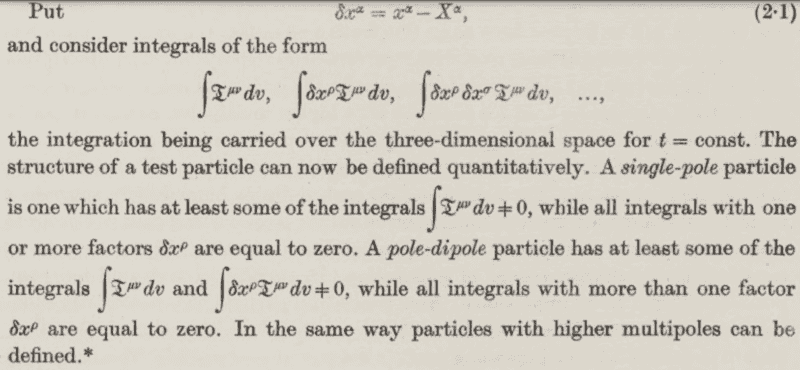# Definition of single-pole and pole-dipole particles

ergospherical
I'd appreciate some clarification of this passage in the paper Spinning test particles in general relativity by Papapetrou,The definition is easy enough to understand, but what's the motivation? ##X^{\alpha}## are the coordinates of points on the worldline whilst ##x^{\alpha}## are presumably arbitrary spacetime coordinates (of points near the worldline).

n.b. ##\mathfrak{T}^{\mu \nu} = \sqrt{-g} T^{\mu \nu}## and\begin{align*}
\nabla_{\nu} T^{\mu \nu} = \partial_{\nu} T^{\mu \nu} + \Gamma^{\nu}_{\sigma \nu} T^{\mu \sigma} + \Gamma^{\mu}_{\sigma \nu} T^{\sigma \nu} &= 0 \\ \\

\implies \dfrac{1}{\sqrt{-g}} \partial_{\nu} \left( \sqrt{-g} T^{\mu \nu} \right) + \Gamma^{\mu}_{\sigma \nu} T^{\sigma \nu} &= 0\\

\partial_{\nu} \left( \sqrt{-g} T^{\mu \nu} \right) + \Gamma^{\mu}_{\sigma \nu} \sqrt{-g} T^{\sigma \nu} &= 0 \\

\partial_{\nu} \mathfrak{T}^{\mu \nu} + \Gamma^{\mu}_{\sigma \nu}\mathfrak{T}^{\sigma \nu} &= 0
\end{align*}

Last edited:

Gold Member
2022 Award
It looks like a Cartesian multipole expansion similar as in electrodynamics, where you have the electric current density ##J^{\mu}## as a source, while here it's of course the energy-momentum tensor as a source of the gravitational field.

BTW: The scans via JSTOR are much better in quality:

https://www.jstor.org/stable/98893

•ergospherical
If beside $\int d^3x \sqrt{-g} T^{\mu\nu} \neq 0$, you have a vanishing higher moments, $\int d^3x \sqrt{-g} \delta x^{\rho}T^{\mu\nu} = 0$ for all $\rho, \mu, \nu$, then the object has no structure, i.e., a single-pole particle. And if the first moment does not vanish, i.e. for some values of the indices, $\int d^3x \sqrt{-g} \delta x^{\rho}T^{\mu\nu} \neq 0$, the object has a structure, i.e., pole-dipole particle. See equations 6,7 and 8 in
•